Courses

# Solutions of Refraction of Light (Page No- 227 & 228) - Physics By Lakhmir Singh, Class 10 Class 10 Notes | EduRev

## Class 10 : Solutions of Refraction of Light (Page No- 227 & 228) - Physics By Lakhmir Singh, Class 10 Class 10 Notes | EduRev

The document Solutions of Refraction of Light (Page No- 227 & 228) - Physics By Lakhmir Singh, Class 10 Class 10 Notes | EduRev is a part of the Class 10 Course Lakhmir Singh & Manjit Kaur Solutions: Class 10 Science.
All you need of Class 10 at this link: Class 10

Page No:227

Question 1: What name is given to the ratio of sine of angle of incidence to the sine of angle of refraction ?

Solution : Refractive index.

Question 2: Write the relation between the angle of incidence and the angle of refraction for a medium.

Solution : Refractive index = sine of the angle of incidence/sine of the angle of refraction.

Page No:228

Question 3: What is the unit of refractive index ?

Solution : Refractive index has no units.

Question 4: Which has higher refractive index : water or glass ?

Solution : Glass has higher refractive index.

Question 5: Refractive indices of carbon disulphide and ethyl alcohol are 1.63 and 1.36 respectively. Which is optically denser ?

Solution : Carbon disulphide is more denser than the ethyl alcohol.

Question 6: The refractive index of diamond is 2.42. What is the meaning of this statement in relation to the speed of light ?

Solution : This means that the ratio of the speed of light in air to the speed of light in diamond is equal to 2.42.

Question 7: If the refractive index for light going from air to diamond be 2.42, what will be the refractive index for light going from diamond to air ?

Solution :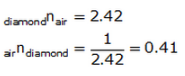Question 8: How is the refractive index of a material related to the speed of light in it ?

Solution : Refractive index of a material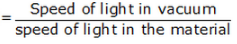Question 9: Fill in the following blank with a suitable word :
When a ray of light goes from air into a clear material, you see the ray bend. How much the ray bends is determined by the………………………. of the material.

Solution : Refractive index.

Question 10: Give three examples of materials that refract light rays. What happens to the speed of light rays when they enter these materials ?

Solution : Three examples of materials that refract light rays are water, glass and diamond. When light rays (travelling in air) enter these materials, their speed decreases.

Question 11: Define Snell’s law of refraction. A ray of light is incident on a glass slab at an angle of incidence of 60°. If the angle of refraction be 32.7°, calculate the refractive index of glass. (Given : sin 60° = 0.866, and sin 32.7° = 540).

Solution : Snell's law: According to the Snell's law of refraction, the ratio of sine of angle of incidence to the sine of angle of refraction is constant for a given pair of media.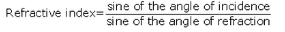Given: Angle of incidence = 60°
Angle of refraction = 32.4°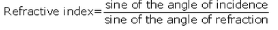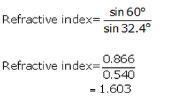Question 12: The speed of light in vacuum and in two different glasses is given in the table below :

 Medium Speed of light VaccumeFlint glasscrown glass 3.00 x 108 m/s1.86 x 108 m/s1.97 x 108 m/s

(a) Calculate the absolute refractive indexes of flint glass and crown glass.
(b) Calculate the relative refractive index for light going from crown glass to flint glass.

Solution :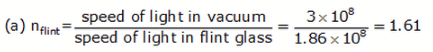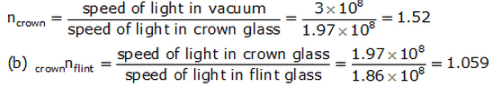Question 13: The speed of light in air is 3 × 108 m/s. In medium X its speed is 2 × 108 m/s and in medium Y the speed of light is 2.5 × 108 m/s. Calculate:
(a) air nx (b) air ny (c) xny

Solution :

Given: Speed of light in air = 3.0x108m/s
Speed of light in medium X = 2.0x108m/s
Speed of light in medium Y = 2.50x108m/s

(a) airnx = ?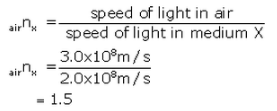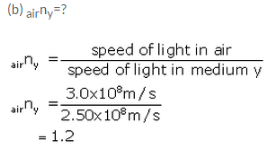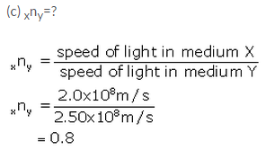Question 14: What is the speed of light in a medium of refractive index 6/5 if its speed in air is 3,00,000 km/s ?

Solution : Refractive index of medium = 6/5 = 1.2
Speed of light in air = 3,00.000km/s

We know that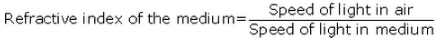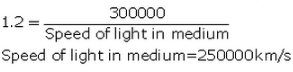Question 15: The refractive index of glass is 1.5. Calculate the speed of light in glass. The speed of light in air is 3.0 × 108ms-1.

Solution : Given:-Refractive index of glass = 1.5
Speed of light in air = 3.0x108m/s

We know that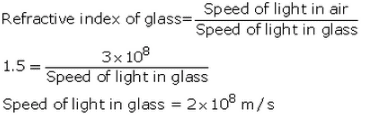Question 16: The speed of light in water is 2.25 × 108 m/s. If the speed of light in vacuum be 3 × 108 m/s, calculate the refractive index of water.

Solution :  Speed of light in vacuum = 3.0x108m/s
Speed of light in water = 2.25 x108m/s
Refractive index of water = ?

We know that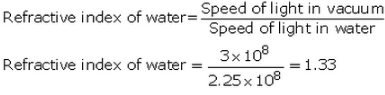Question 17: Light enters from air into diamond which has a refractive index of 2.42. Calculate the speed of light in diamond. The speed of light in air is 3.0 × 108 ms-1.

Solution : Given:- Refractive index of diamond = 2.42
Speed of light in air = 3.0x108m/s We know that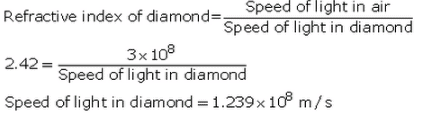Question 18: (a) State and explain the laws of refraction of light with the help of a labelled diagram.
(b) What is meant by the refractive index of a substance ?
(c) Light travels through air at 300 million ms-1. On entering water it slows down to 225 million ms-1

Calculate the refractive index of water.

Solution :

(a) Law of refraction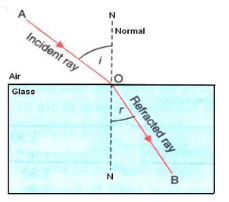First law: According to the first law of refraction, the incident ray. the refracted ray and the normal at the point of incidence, all lie in the same plane.

Second law: According to the second law of refraction, the ratio of the sine of angle of Incidence to the sine of angle of refraction is constant for a given pair of media.

(b) Refractive index of substance: The ratio of speed of light In vacuum to the speed of light In a medium. Is called the refractive index of that medium.

(c) Speed of light in air = 300 milion m/sec

Speed of light in water = 225 milion m/sec

We know that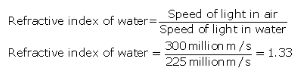Page No:229

Question 29: The following table gives the refractive indices of a few media :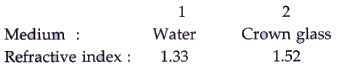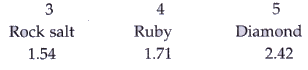Use this table to give an example of :
(i) a medium pair so that light speeds up when it goes from one of these medium to another.
(ii) a medium pair so that light slows down when it goes from one of these medium to another.

Solution :

(i) Crown glass to water.
(ii) Water to diamond.

Question 30: Refractive indices of four media A, B, C and D are given below :

 Medium Refractive index A 1.33 B 1.44 C 1.52 D 1.65

In which of these four media is the speed of light (i) maximum, and (ii) minimum ?

Solution :

(i) A (It has least refractive index).
(ii) D (It has highest refractive index).

Page No:239

Question 1: Name the lens which can concentrate sun’s rays to a point and burn a hole in a piece of paper.

Solution : Converging lens.

Question 2: Give the usual name for the following :

A point inside a lens through which the light passes undeviated.

Solution : Optical center.

Question 3: A 1 cm high object is placed at a distance of 2ƒ from a convex lens. What is the height of the image formed ?

Solution : 1 cm (same as the height of the object).

Question 4: If the image formed by a convex lens is of the same size as that of the object, what is the position of the image with respect to the lens ?

Solution : At 2F (At twice the focal length).

Question 5: If an object is placed at the focus of a convex lens, where is the image formed ?

Solution : The image is formed at infinity (at very large distance).

Question 6: Where should an object be placed in order to use a convex lens as a magnifying glass ?

Solution : Object should be placed at a distance less than focal length.

Question 7: Where should an object be placed in front of a convex lens so as to obtain its virtual, erect and magnified image ?

Solution : The object should be placed within focus.

Question 8: Where should an object be placed in front of a convex lens so as to obtain its real, inverted and magnified image ?

Solution : Object should be placed between f and 2f.

Page No:240

Question 9: For what position of an object a real, diminished image is formed by a convex lens ?

Solution : Beyond 2F.

Question 10: If an object is at a considerable distance (or infinity) in front of a convex lens, where is the image formed ?

Solution : At focus F.

Question 11: Draw the given diagram in your answer book and complete it for the path of a ray of light after passing through the lens.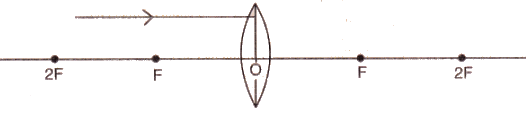Solution :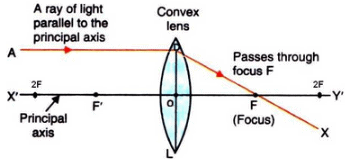Question 12: What type of lens would you use as a magnifying glass ? How close must the object be to the lens ?

Solution : Convex lens

The object must be withing the focus of the lens.

Question 13: Name two factors on which the focal length of a lens depends.

Solution : Focal length of a lens depends on the refractive index of the glass from which it is made, and on the curvature of its two surfaces.

Question 14: State any two uses of convex lenses.

Solution : Two uses of convex lenses:-
1. As a magnifying glass.
2. For making a simple camera.

Question 15: Fill in the following blanks with suitable words :
(a) Parallel rays of light are refracted by a convex lens to a point called the……….
(b) The image in a convex lens depends upon the distance of the…………. from the lens.

Solution :
a) focus.
b) object.

Question 16: What is a lens ? Distinguish between a convex lens and a concave lens. Which of the two is a converging lens : convex lens or concave lens ?

Solution : A lens is a piece of transparent glass bound by two spherical surfaces.
A convex lens is thicker at the middle as compared to the edges; while a concave lens is thicker at the edges as compared to the middle.
Convex lens is converging lens.

Question 17: (a) Explain with the help of a diagram, why the convex lens is also called a converging lens.
(b) Define principal axis, principal focus and focal length of a convex lens.

Solution : (a) A convex lens also known as converging type because it converges a parallel beam of light rays passing through it.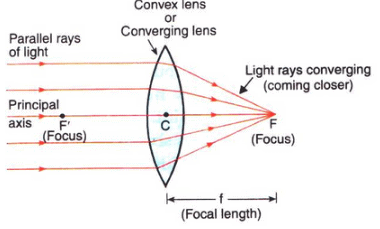(b) Principle axis: The principal axis of a lens is a line passing throught the optical centre of the lens and perpendicular to both the faces of the lens.
Principle focus: The principal focus of a convex lens is a point on its principal axis to which light rays parallel to the principal axis converge after passing through the lens.
Focal length: The distance of the principle focus from the optical center of a lens is called its focal length.

Question 18: (a) Explain with the help of a diagram, why the concave lens is also called a diverging lens.
(b) Define the principal focus of a concave lens.

Solution : (a) A concave lens is known as diverging lens because it diverges the parallel rays of light passing through it.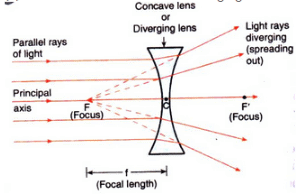(b) The principal focus of a concave lens is a point on its principal axis from which light rays, originally parallel to the axis, appear to diverge after passing through the lens.

Question 19: Draw a ray diagram to show the formation of a real magnified image by a convex lens. (In your sketch the position of object and image with respect to the principal focus of lens should be shown clearly).

Solution : Formation of real magnified image by a convex lens.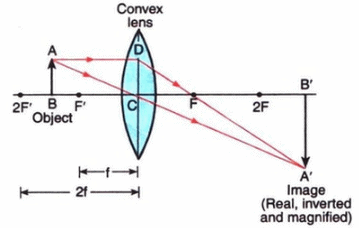Question 20: Describe with the help of a ray-diagram, the formation of image of a finite object placed in front of a convex lens between ƒ and 2ƒ Give two characteristics of the image so formed.

Solution : If object is placed in between f and 2f, the image will form on the other side of the lens beyond 2f as shown below.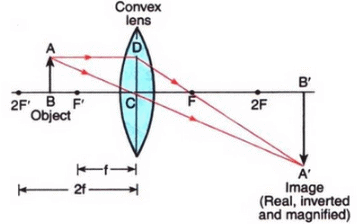Characteristics of image formed:
Image formed is real and inverted.
Image formed is magnified.

Question 21: Describe with the help of a ray diagram the nature, size and position of the image formed when an object is placed in front of a convex lens between focus and optical centre. State three characteristics of the image formed.

Solution : In the diagram, the object is placed in front of a convex lens between focus and optical centre. The image is formed on the same side as the object as shown below.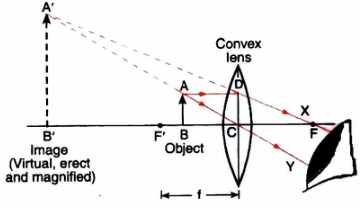Characteristics of image formed:
Image is virtual and erect.
Image is larger than the object
Image is formed behind the object.

Question 22: An object is placed at a distance equal to 2ƒ in front of a convex lens. Draw a labelled ray diagram to show the formation of image. State two characteristics of the image formed.

Solution :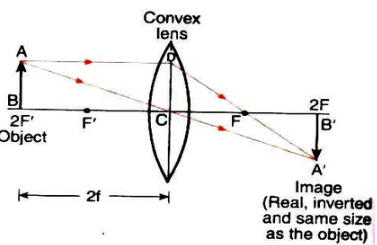Characteristic of image formed:
Image formed is real and inverted.
Image is of same size as the object

Question 23: Describe with the help of a ray-diagram, the size, nature and position of the image formed by a convex lens when an object is placed beyond 2f in front of the lens.

Solution : When an object is placed beyond 2f in front of a convex lens, then the image formed is between f and 2f on the other side of the lens, it is real, inverted and smaller than the object.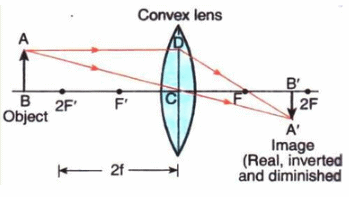Question 24: Describe with the help of a ray diagram the nature, size and position of the image formed when an object is placed at infinity (considerable distance) in front of a convex lens. State three characteristics of the image so formed.

Solution : When an object is placed at infinity in front of a convex lens, the image is formed at the focus on the other side of the lens.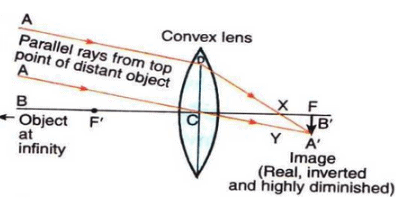Characteristics of image formed:
Image is real.
Image is inverted.
Image is highly diminished.

Question 25: (a) What type of lens is shown in the diagram on the right ? What will happen to the parallel rays of light ? Show by completing the ray diagram.
(b) Your eye contains a convex lens. Why is it unwise to look at the sun ?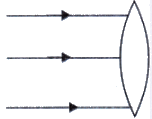Solution : (a) The lens shown in convex. the parallel rays will converge to a point called focus (F).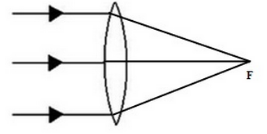(b) It is unwise to look at the sun because the convex lens focusses a lot of sun rays into our eyes and this may damage them.

Question 26: Where must the object be placed for the image formed by a converging lens to be :
(a) real, inverted and smaller than the object ?
(b) real, inverted and same size as the object ?
(c) real, inverted and larger than the object ?
(d) virtual, upright and larger than the object ?

Solution : a) Beyond 2F
b) At 2F
c) Between F and 2F
d) Between F and optical centre

Question 27: Draw a diagram to show how a converging lens held close to the eye acts as a magnifying glass. Why is it usual to choose a lens of short focal length for this purpose rather than one of long focal length ?

Solution : Converging lens as a magnifying glass: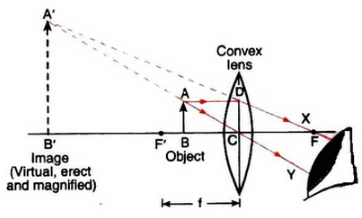It is usual to choose a lens of short focal length for this purpose rather than one of long focal length because smaller the focal length of a convex lens, greater will be its magnifying power.

Question 28: How could you find the focal length of a convex lens rapidly but approximately ?

Solution : To determine the focal length of a convex lens, we put the convex lens in a holder and keep it in front of a distant object like a window or tree, so that the rays coming from the window pass through it. A cardboard screen is put behind the lens. We change the distance of the screen from the convex lens until a clear inverted image of the window is formed on the screen. Measure the distance of the screen from the lens with a scale. This distance will be the focal length of convex lens.

Question 29: (a) With the help of a labelled diagram explain how a convex lens converges a beam of parallel light rays.
Mark the principal axis, optical centre, principal focus and focal length of the convex lens on the diagram.
(b) State whether convex lens has a real focus or a virtual focus.
(c) List some things that convex lens and concave mirror have in common.

Solution : (a) When a beam of light rays parallel to one another and also to the principal axis of the convex lens fall on the lens, the incident rays pass through the lens and get refracted according to the laws of refraction. All the rays, after passing through the lens, converge at the same point F (focus) on the other side of the lens.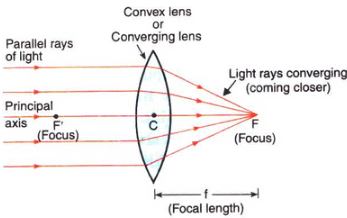(b) A convex lens has a real focus.
(c) Both, convex lens and concave mirror, converge parallel rays of light coming from infinity (parallel to the principal axis) at the focus.

Page No:241

Question 30: (a) With the help of a labelled diagram, explain how a concave lens diverges a beam of parallel light rays.
Mark the principal axis, optical centre, principal focus and focal length of the concave lens on the diagram.
(b) State whether concave lens has a real focus or a virtual focus.
(c) List some things that concave lens and convex mirror have in common.

Solution : (a)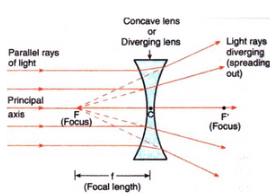Figure — A concave lens diverges (spreads out) a parallel beam of light rays.

When a beam of light rays parallel to one another and also to the principal axis of the concave lens fall on the lens, the incident rays pass through the lens and get refracted according to the laws of refraction. All the rays spread out after passing through the lens. These diverging rays when produced backwards appear to meet at a point F (focus) on the left side of the lens.
(b) A concave lens has a virtual focus.
(c) Both, concave lens and convex mirror, diverge parallel rays of light coming from infinity (parallel to the principal axis).

Question 31: Draw ray diagrams to represent the nature, position and relative size of the image formed by a convex lens for the object placed :
(a) at 2F1,
(b) between F1and the optical centre O of the lens.
Which of the above two cases shows the use of convex lens as a magnifying glass ? Give reasons for your choice.

Solution :

(a) object at 2F1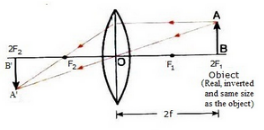(b) Object between F1 and the optical centre O of the lens :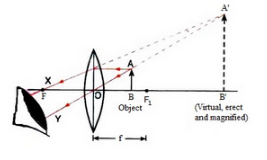The case when object is between F1 and the optical centre O of the lens shows the use of convex lens as a magnifying glass. This is because here the image formed is erect and magnified.

Question 32: (a) An object is placed well outside the principal focus of a convex lens. Draw a ray diagram to show how
the image is formed, and say whether the image is real or virtual.
(b) What is the effect on the size and position of the image of moving the object (i) towards the lens, and (ii) away from the lens ?

Solution : (a)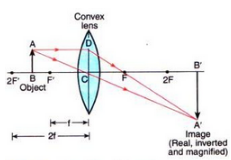Figure — Formation of image by a convex lens when the object is placed between F' and 2F" (or between f and 2f)

(b) (i) If object is moved towards the lens, the image size will keep on increasing till the object reaches focus. After that, the size decreases but the image remains magnified. The image keeps movingvaway from the lens (on the opposite side of the lens) till the object reaches focus; after that the image is formed on the same side of the lens as the object.
(ii) If object is moved away from the lens, the size will keep on decreasing and the image keeps on shifting towards the lens.

Question 33: (a) Explain what is meant by a virtual, magnified image.
(b) Draw a ray diagram to show the formation of a virtual magnified image of an object by a convex lens. In your diagram, the position of object and image with respect to the principal focus should be shown clearly.
(c) Three convex lenses are available having focal lengths of 4 cm, 40 cm and 4 m respectively. Which one would you choose as a magnifying glass and why ?

Solution : (a) A virtual magnified image is the one which cannot be taken on a screen and whose size is larger than that of the object.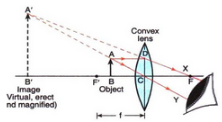Figure - Formation. of image by a convex lens when the object is placed between its optical center (C) and focus (F).

(c) Convex lens having a cm focal length - because it wil produce greatest magnification

Question 34: (a) Explain why, a real image can be projected on a screen but a virtual image cannot.
(b) Draw a ray diagram to show the formation of a real diminished image of an object by a convex lens. In your diagram, the position of object and image with respect to the principal focus should be shown clearly.
(c) Name one simple optical instrument in which the above arrangement of convex lens is used.

Solution : a) A real image can be projected on a screen but a virtual image cannot because a real image is formed when light rays coming from an object actually meet at a point after refraction through a lens while a virtual image is formed when light rays coming from an object only appear to meet at a point when produced backwards (but do not actually meet) after refraction through a lens.

(b)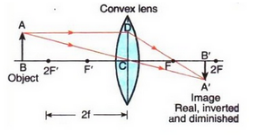(c) A simple Camera

Page No:242

Question 46: A lens of focal length 12 cm forms an erect image three times the size of the object. The distance between the object and image is :
(a) 8 cm
(b) 16 cm
(c) 24 cm
(d) 36 cm

Solution :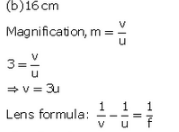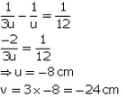Distance between object and im age= u-v= -8-(-24) = - 8 + 2 4 = 16 cm

Question 47: If an object is placed 21 cm from a converging lens, the image formed is slightly smaller than the object. If the object is placed 19 cm from the lens, the image formed is slightly larger than the object. The approximate focal length of the lens is :
(a) 5 cm
(b) 10 cm
(c) 18 cm
(d) 20 cm

Solution : (b) 10 cm
The image is slightly smaller than the object when the object lies beyond 2f; and the image is slightly larger than the object when the object between f and 2f. This means that between 21 cm and 19 cm lies 2f. Out of the given options, 20 cm lies between 21 cm and 19 cm.
So, 2f = 20 cm.
f = 10 cm.

Question 48: An object is placed at the following distances from a convex lens of focal length 15 cm :
(a) 35 cm
(b) 30 cm
(c) 20 cm
(d) 10 cm
Which position of the object will produce :
(i) a magnified real image ?
(ii) a magnified virtual image ?
(iii) a diminished real image ?
(iv) an image of same size as the object ?

Solution : Here, f = 15cm and 2f = 30cm
(i) 20 cm (Because a magnified real image is formed when the object is placed between f and 2f).
(ii) 10cm (Because a magnified virtual image is formed when the object is placed between f and the lens).
(iii) 35cm (Because a diminished real image is formed when the object is placed beyonf 2f).
(iv) 30cm (Because an image of same size as the object is formed when the object is placed at 2f).

Question 49: When an object is placed at a distance of 36 cm from a convex lens, an image of the same size as the object is formed. What will be the nature of image formed when the object is placed at a distance of:
(a) 10 cm from the lens ? (b) 20 cm from the lens ?

Solution : Here, 2f = 36 cm, f = 18 cm.
(a) When the object is placed at a distance of 10 cm from the lens, the object lies within the focus. Hence, the image formed is virtual, erect and magnified.
(b) When the object is placed at a distance of 20 cm from the lens, the object lies between f and 2f. Hence, the image formed is real, inverted and magnified.

Question 50: (a) Draw a diagram to show how a converging lens focusses parallel rays of light.
(b) How would you alter the above diagram to show how a converging lens can produce a beam of parallel rays of light.

Solution : (a) A converging lens focusses parallel ray of light as shown below: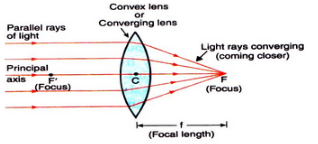Figure — A convex lens converges (brings closer) a parallel beam of light rays to a point F on its other side (right side).

(b) Place a source of light at the focus of the converging lens.

Offer running on EduRev: Apply code STAYHOME200 to get INR 200 off on our premium plan EduRev Infinity!

61 docs

,

,

,

,

,

,

,

,

,

,

,

,

,

,

,

,

,

,

,

,

,

,

,

,

;